# Real World Integer Subtraction

Real World Integer Subtraction Visual Number Talk introduced through the context of rising and falling water levels compared to sea level.

## In This Set of Visual Number Talk Prompts…

Students will explore integer subtraction through a real world contextual situation involving rising and falling water levels compared to sea level.

## String of Related Problems

Present the following subtraction sentences one at a time. Encourage students to use an open number line to defend their answer.

The visuals provided here explore the first two subtraction sentences, however Day 3 of the Rising Water unit provides an additional four (4) visual number talk prompts.

12 – 8

8 – 12

5 – (-1)

-1 – (5)

-3 – (-7)

-7 – (-3)

Facilitator Note: The big idea here is difference. We are looking at the difference between these two values. However, the order matters. Unlike with addition, subtraction does not follow the commutative property. Is the minuend more or less than the subtrahend?

Minuend − Subtrahend = difference

When the minuend is greater than the subtrahend, the difference is positive. When the minuend is less than the subtrahend, the difference is negative. Seeing these values on the number line should be helpful to solidify this generalization.

## Visual Math Talk Prompt #1

Students will be prompted with:

Construct a subtraction sentence to represent and determine the difference in water height from last year to this year. Use a number line to defend your answer.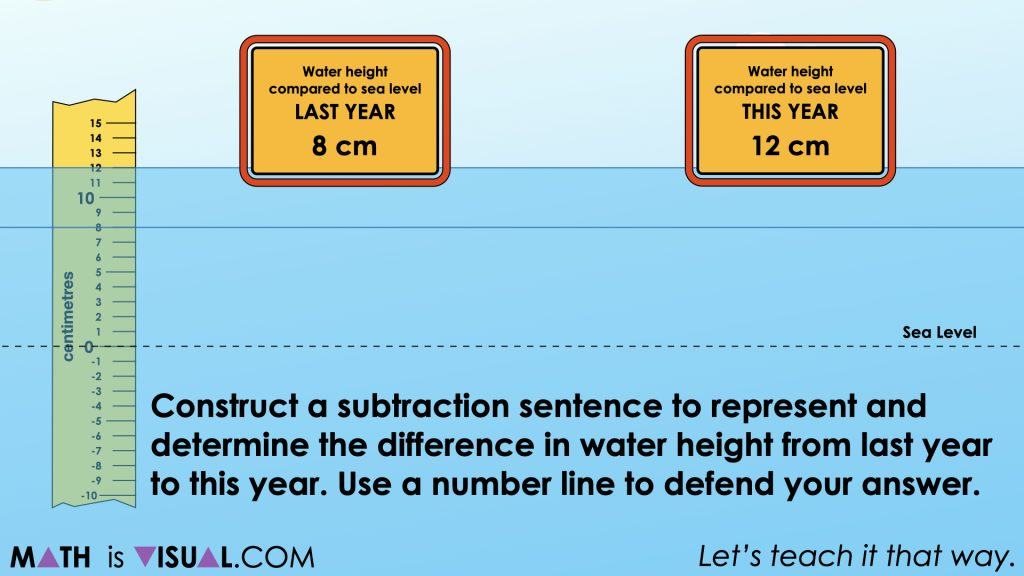This context builds on the work students have already engaged in over the first two days of the Rising Water 6-Day Problem Based Unit on the Make Math Moments website involving a glacier melting and increasing water levels over time.

In this case, we are emerging the idea of subtraction as a difference between the water height this year compared to last year.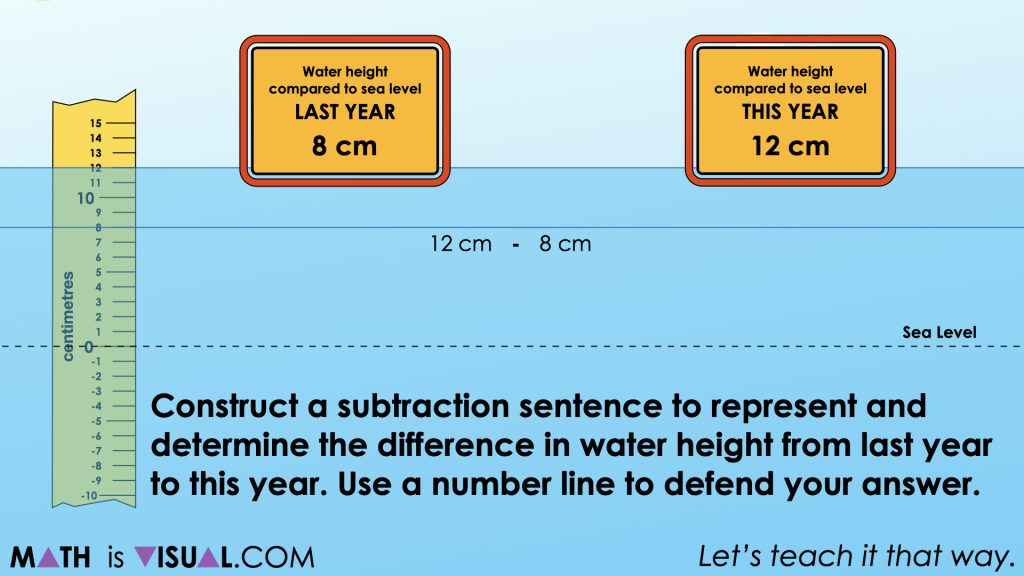In the first prompt in this string of related problems, we provide students with a higher water level this year than last to result in a positive result. In later prompts in this string, we will do the opposite to result in negative differences.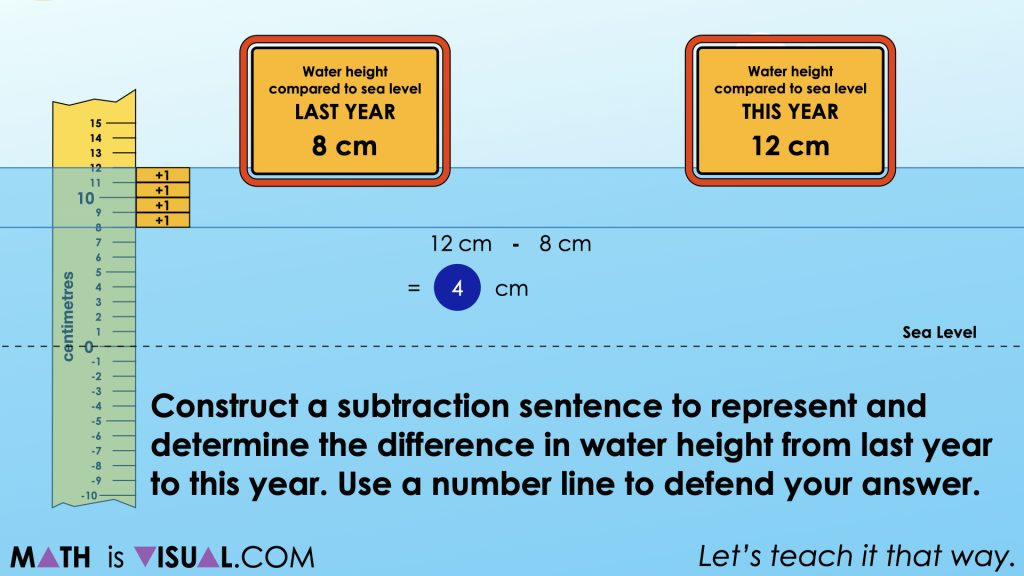Although students are able to solve this problem however they choose, we will explicitly share a linear model as a tool to represent thinking so we can leverage that same tool once negative differences come into play later in the string.

## Visual Math Talk Prompt #2

Students will be prompted with:

Construct a subtraction sentence to represent and determine the difference in water height from last year to this year. Use a number line to defend your answer.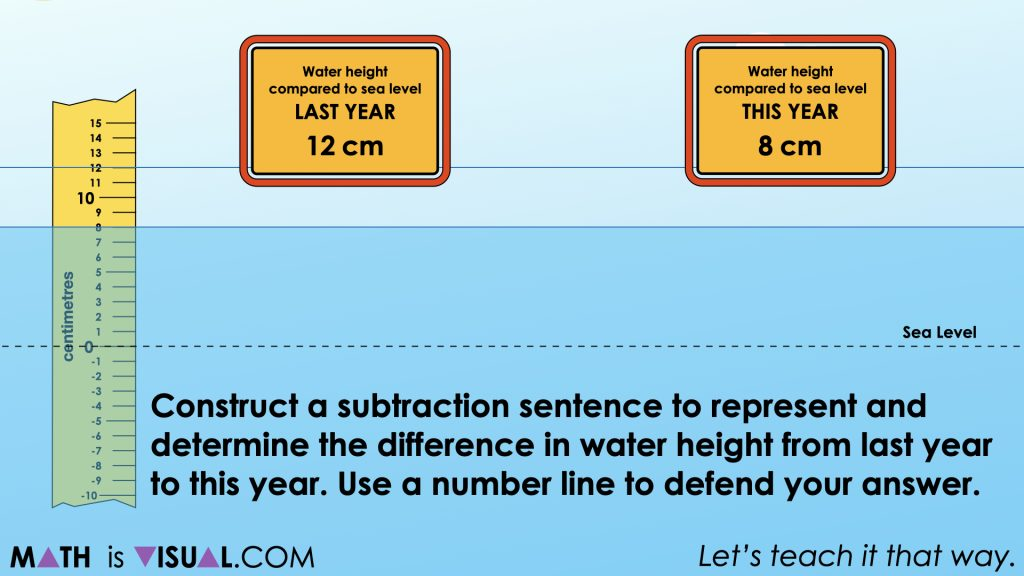In the second visual number talk prompt from this string of related problems, we reverse the water heights so that the water level is decreasing from last year to this year. The result is that the difference will be a negative measure and we can explicitly show this on the number line to again promote this model as a tool for thinking as well as a tool to represent thinking.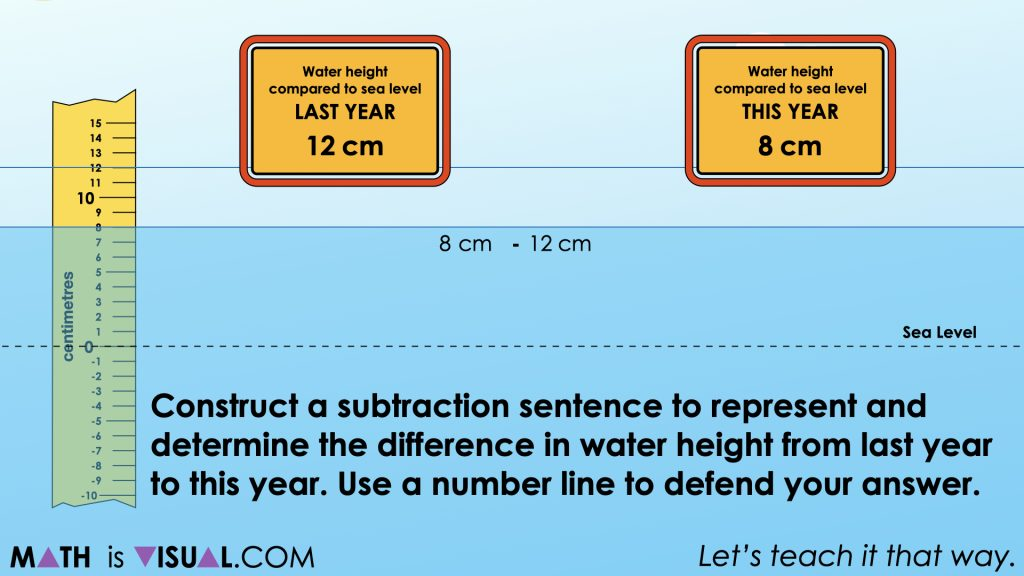While it might seem obvious that the result must be a negative number since the water level has decreased from last year to this year, we are again leveraging the number line to build our confidence with the model to help us when the situation becomes more complex in future problems in the string.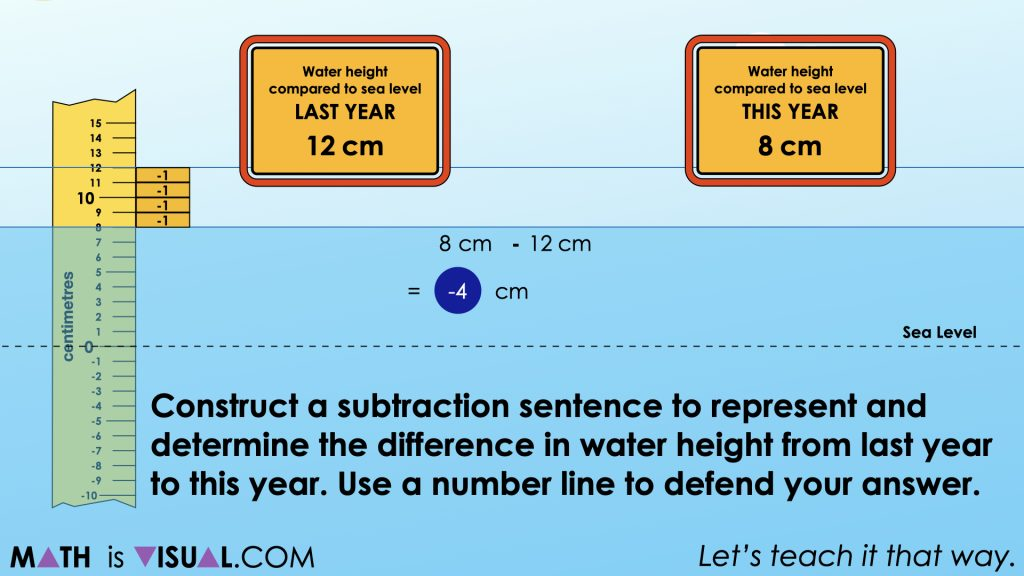## Want to Explore These Concepts & Skills Further?

Four (4) additional number talk prompts are available in Day 3 of the Rising Water problem based math unit that you can dive into now.

Why not start from the beginning of this contextual 5-day unit of real world lessons from the Make Math Moments Problem Based Units page.

Did you use this in your classroom or at home? How’d it go? Post in the comments!

Math IS Visual. Let’s teach it that way.